### Coupled oscillations, other systems

#### Problem:

Two pendula, each of which consists of a weightless rigid rod length of L and a mass m, are connected at their midpoints by a spring with spring constant k.  Consider only small displacements from equilibrium.(a)  What are the frequencies of the normal modes of this system?  Briefly describe these modes.
(b)  At t = 0 the right pendulum is displaced by an angle θ to the right while the left pendulum remains vertical.  Both pendula are released from rest.  Describe the subsequent motion.

Solution:

• Concept:
Small oscillations:
L = ½∑ij[Tij(dqi/dt)(dqj/dt) - kijqiqj]  with Tij = Tji,  kij = kji
Solutions of the form qj = Re(Ajeiωt) can be found.
For a particular frequency ωα we solve
j[kij - ωα2Tij]A = 0
to find the A.  The most general solution for each coordinate qj is α sum of simple harmonic oscillations in all of the frequencies ωα.
qj = Re∑α(CαAexp(iωαt)).
• Reasoning:
We are asked to solve for the motion of two coupled pendula.
• Details of the calculation:
(a)  Call the left pendulum 1 and the right pendulum 2.  Let θi denote a small angular displacement of pendulum i to the right.
Small angle approximation:  sinθ = θ, cosθ = 1 in the equations of motion.
Define x1 = Lθ½,  x2 = Lθ2/2
τ1 = Iα1 = mL2d2θ1/dt2 = -mgLθ1 - kL21 - θ2)/4
τ2 = Iα2 = mL2d2θ2/dt2 = -mgLθ2 - kL22 - θ2)/4
Equations of motion:
d2θ1/dt2 = -(g/L)θ1 - (k/m)(θ1 - θ2)/4
d2θ2/dt2 = -(g/L)θ2 - (k/m)(θ2 - θ1)/4
Try solutions of the form θi = θi0 exp(iωt).  Then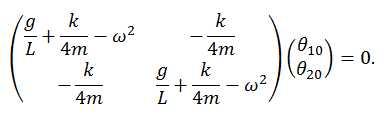Solve for ω by setting the determinant of the matrix equal to zero.
ω2 = (g/L + k/(4m)) ± k/(4m).

ω12 = g/L,  θ10 = θ20,  the pendula oscillate in phase.
ω22 = g/L + k/(2m),   θ10 = -θ20, the pendula oscillate 180o out of phase.

(b)  Most general solution:
θ1(t) = Re(C1exp(iω1t) + C2exp(iω2t))
θ2(t) = Re(C1exp(iω1t) - C2expi(ω2t))
1(t)/dt = Re(iω1C1exp(iω1t) + iω2C2exp(iω2t))
2(t)/dt = Re(iω1C1exp(iω1t) - iω2C2expi(ω2t))
Initial conditions at t = 0:
Re(C1 + C2) = 0,   Re(C1 - C2) = θ20,  Re(C1) = - Re(C2) = θ20/2.
Im(ω1C1+ ω2C2) = 0, Im(ω1C1+ ω2C2),  Im(C1) = Im(C2) = 0.

θ1(t) = (θ20/2)(cos(ω1t) - cos(ω2t))
θ2(t) = (θ20/2)(cos(ω1t) + cos(ω2t))

#### Problem:

A rigid uniform bar of mass M and length L is supported in equilibrium in a horizontal position by two massless springs attached at each end.  The identical springs have spring constant k.  The motion of the center of mass is constrained to move parallel to the vertical x-axis.  Furthermore, the motion of the bar is constrained to lie in the xz plane.  Let x1 and x2 be the departures of the two ends from their equilibrium positions, as shown.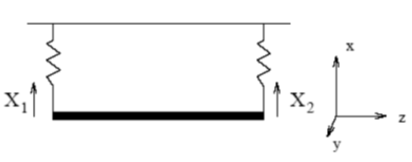(a)  Show that the moment of inertia for a bar about the y-axis through its center of mass is ML2/12.
(b)  Construct the Lagrangian for the bar-spring arrangement assuming only small deviations from equilibrium.
(c)  Calculate the vibrational frequencies of the normal modes for small amplitude oscillations.
(d)  Describe the normal modes of oscillations.

Solution:

• Concepts:
Small oscillations, coupled oscillations, normal modes
• Reasoning:
L = ½∑ij[Tij(dqi/dt)(dqj/dt) - kijqiqj]  with Tij = Tji,  kij = kji
Solutions of the form qj = Re(Ajeiωt) can be found.  We can find the ω2 from det(kij - ω2Tij) = 0.
• Details of the calculation:
(a)  Put the origin of the coordinate system at the CM.
I = ∫r2 dm with dm with dm = (M/L)z.
I = 2∫0L/2 z2 (M/L) dz =2(M/L)[z3/3]0L/2 = ML2/12.
(b)  L = Ktr + Krot - U.   Let x1 and x2 be the displacements from equilibrium.
Ktr = ½M(dxCM/dt)2 = (1/8)M(dx1/dt + dx2/dt)2.
Krot = ½I(d/θdt)2 =½(ML2/12)[(dx1/dt - dx2/dt)/L]2 = (½4)M(dx1/dt - dx2/dt)2.
We use the small angle approximation, sinθ = θ = (x2 - x1)/L,  cosθ = 1 - θ2/2.
We have elastic and gravitational potential energy.
Usp = ½k(x1 - x0)2 + ½k(x2 - x0) 2,  Ug = ½ Mg(x1 + x2)
U = ½kx12 +½kx22 + kx02  - kx0x1 - kx0x2 + ½Mgx1 + ½Mgx2
Since kx0 = ½Mg we can write U = ½kx12 + ½kx22, and
L = (1/6)M[(dx1/dt)2 + (dx2/dt)2] + (1/6)M(dx1/dt)(dx2/dt) - ½kx12 - ½kx22.
(c)  We can write
L = ½∑ij[Tij(dqi/dt)(dqj/dt) - kijqiqj]  with Tij = Tji,  kij = kji
Here T11 = T22 = M/3, T12 = T21 = M/6,  k11 = k22 = k,  k12 = k21 = 0.
Solutions of the form qj = Re(Ajeiωt) can be found.  We need
det(kij2Tij) = 0.ω2 = 4k/M ± 2k/M,  ω- = (2k/M)½,  ω+ = (6k/M)½
(d)  The normal mode corresponding to ω- has the bar moving up and down while remaining horizontal.  That corresponding to ω+ has the center of mass of the bar remain stationary while the two ends oscillate up and down exactly out of phase.

#### Problem:

A uniform horizontal rectangular plate (mass M, length L, width W) rests with its corners on four similar vertical springs with spring constant k.  Find the normal modes of vibration and prove that their frequencies are in the ratio 1 : √3 : √3.
Solution:

• Concepts:
Small oscillations, normal modes
• Reasoning:
We are asked to find the 3 normal modes of the plate.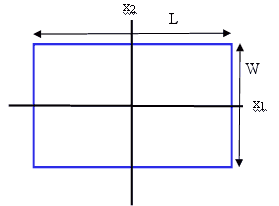Assume that the CM of the plate is restricted to move along a vertical line.
The symmetry of the setup implies that the three normal modes are:
(a)  The plate moves up and down and does not rotate.
(b)  The plate rotates about the line x2, the CM is fixed.
(c)  The plate rotates about the line x1, The CM is fixed.
• Details of the calculation:
(a)  T = ½M(dz/dt)2.  Here z is the displacement from its equilibrium position in the gravitational field.
U = ½4kz2,  d2z/dt2 = -4(k/m)z,  ωa2 = 4k/M.
(b)  T = ½Ib(dθ/dt)2,  θ = 2z/L for small oscillations.  U = ½4k(L2/4)θ2.
Ib = 2W∫0L/2ρ(x)x2dx = 2Wρ(x)(L/2)3/3 = 2W(M/(LW))(L/2)3/3 = ML2/12.
Ib = ML2/12.
d2θ/dt2 = -12 (k/m)θ,  ωb2 = 12(k/m),  ωb = (3)½ωa.
(c)  T = ½Ic(dΦ/dt)2,  Φ = 2z/W for small oscillations.  U = ½4k(W2/4)Φ2.
Ic = MW2/12.
d2Φ/dt2 = -12 (k/m)Φ,  ωc2 = 12(k/m),  ωc = (3)½ωa.

#### Problem:

Phonons are quantized lattice vibrations, and many aspects of these excitations can be understood in terms of simple mode counting.
(a)  Estimate the number of phonon modes in 1 cm3 of a crystalline material with an inter-atomic spacing of 2 Angstrom.
(b)  Assuming that in thermal equilibrium each phonon mode has kBT of energy, give a numerical estimate of the heat capacity ΔE/ΔT of this 1 cm3 of material, in [J/K].

Solution:

• Concepts:
Normal modes of coupled oscillators
• Reasoning:
We are asked to estimate the number of normal modes of a large number of coupled harmonic oscillators.
• Details of the calculation:
(a) We have N atoms, N coupled oscillators.  We therefore have 3N normal modes.  (We may want to neglect translation and rotation of the crystal as a whole, but since N is so large, it does not make any difference.)
N = n3, n = 10-2m/(2*10-10m) = 5*107.  N = 1.25*1023.
(b)  kB = 1.380658 * 10-23 J/K.
E = 3NkBT,  ΔE/ΔT = 3NkB = 5.19 J/K  for the 1 cm3 piece of material.
This is a reasonable number.  For water the specific heat is 4.186 J/(g K).

#### Problem:

A naive model of a solid is that of a bunch of balls (atoms) connected by springs (bound by inter-atomic potentials which can be approximated by harmonic potentials near equilibrium).  If each inter-atomic spring has spring constant k, you can relate this microscopic value to the macroscopically measurable value of Young's modulus, Y.  Young's modulus is the ratio of stress (F/A, or applied force, F, perpendicular to the cross-sectional area, A, of a bar of material per unit cross-sectional area), to strain (ΔL/L, or the fractional change in length of the bar of material); thus, Y = (F/A)/(ΔL/L).  Evaluate k for the inter-atomic springs of aluminum, which has a Young's modulus of 70 GPa.  Assume that the aluminum atoms are arranged in a simple cubic lattice (they are really face-centered cubic); you can determine the inter-atomic spacing by knowing that the density of aluminum is 2.70 g/cm3 and that a mole of aluminum has a mass of 27 g.  Express your result for k in SI units.

Solution:

• Concepts:
Hooke's law, general physics
• Reasoning:
We are asked to use general physics principles to evaluate a simple model of a solid.
• Details of the calculation:
Assume we have N atoms in a cube of volume 1 m3.   Let n = N/m3.  Then we have N(2/3) atoms on each 1 m2 surface.  Assume each atom is acted of by a force f perpendicular to the surface and pointing outward, f = (F/A)/n(2/3).  Each spring in a chain of atoms perpendicular to the surface is stretched by an amount x = f/k, the change in length of the chain is ΔL/L = n(1/3)f/k.
Therefore Y = fn(2/3)/( n(1/3)f/k) = kn(1/3)
n = (2.7 g/cm3)*(106 cm3/m3)*(6.02*1023 atoms/mole)*1 mole/27 g) = 6.02*1028 atoms/m3.
k = (70*109/3.92*109) N/m  = 17.9 N/m.
(This yields a reasonable vibrational frequency of ω = (k/m)½ = 2*1013/s for out of phase motion.)

#### Problem:

A pendulum consisting of a mass m and a weightless string of length l is mounted on a mass M, which in turn slides on a support without friction and is attached to a horizontal spring with force constant k, as seen in the diagram.  There is a slot in the support in order that the pendulum may swing freely.
(a)  Set up Lagrange's equations.
(b)  Find the normal mode frequencies for small oscillations. What are those frequencies to zeroth order in m/M, when  m << M?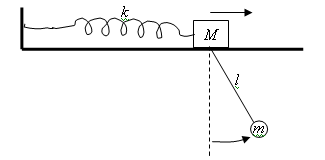Solution:

• Concepts:
Lagrangian Mechanics, coupled oscillations
• Reasoning:
We are asked to write down and solve Lagrange's equations.
• Details of the calculation:
(a) T = ½M(dX/dt)2 + ½m[(dx/dt)2 + (dy/dt)2],  x = X + ℓ sinθ, y = -ℓcosθ.
T = ½M(dX/dt)2 + ½m[(dX/dt)2 + ℓ2(dθ/dt)2 + 2(dX/dt)(dθ/dt)ℓcosθ].
U = -mgℓcosθ + ½kX2
L = T - U = ½(M + m)(dX/dt)2 + ½m[ℓ2(dθ/dt)2 + 2(dX/dt)(dθ/dt)ℓcosθ] + mgℓcosθ - ½kX2
We have two generalized coordinates, X and θ.  Lagrange's equations are
d/dt ∂L/∂(dX/dt) - ∂L/∂X = 0,  d/dt ∂L/∂(dθ/dt) - ∂L/∂θ = 0.
1st equation:  (M + m)d2X/dt2 + md2θ/dt2ℓcosθ - m(dθ/dt)2ℓsinθ = -kX.
2nd equation: ℓ2d2θ/dt2 + d2X/dt2ℓcosθ = -gℓ sinθ.

(b)  Assume X and θ are small quantities, and in the equations of motion keep only terms to first order in small quantities.  Then we have, using sinθ ~ θ, cosθ ~ 1.
1st equation:  (M + m)d2X/dt2 + mℓd2θ/dt2  = -kX.
2nd equation: d2θ/dt2 + (1/ℓ)d2X/dt2  = -(g/ℓ)θ.
We obtain the same equations if we make the small displacements approximation earlier and keep only terms up to second order in the Lagrangian.
Try solutions X = Aexp(iωt),  θ = (B/ℓ)exp(iωt),  then
2(M + m)A - ω2mB = -kA,  -ω2B - ω2A = -(g/ℓ)B.
We solve this system of equations by setting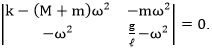ω4 - ω2(k/M + (g/ℓ)(1 + m/M)) + kg/(ℓM) = 0.
ω2 = (k/M + (g/ℓ)(1 + m/M))/2 ± ½((k/M + (g/ℓ)(1 + m/M))2 - 4kg/(ℓM))½.
ω+2 = (k/M + (g/ℓ)(1 + m/M))/2 + ½((k/M + (g/ℓ)(1 + m/M))2 - 4kg/(ℓM))½.
ω-2 = (k/M + (g/ℓ)(1 + m/M))/2 - ½((k/M + (g/ℓ)(1 + m/M))2 - 4kg/(ℓM))½.
The frequencies for small oscillations are ω+ and ω-.
[For the relative displacements we have B =  Aω2/((g/l) - ω2).  ω+2 > g/l,  so A and B have opposite signs for this mode.]

As m --> 0, ω+ --> (k/M)½, ω- = (g/ℓ)½.  The modes effectively decouple.  The small mass m does no longer influence the motion of the big mass M.  When M oscillates with frequency (k/M)½, m becomes a driven harmonic oscillator without damping (for small oscillations).  The most general solution is a superposition of oscillations with the driving frequency and oscillations with the natural frequency of the oscillator.